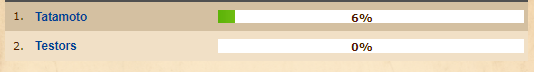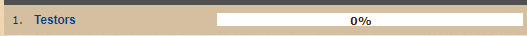# AACQuest Maker have a problem

#### asdzx123

##### Member
Hello
Why when changing the limit to number 1, the first player does not appear at the top, on the contrary, the second player appears at the top, where is the situation?

\$players = \$SQL->query('SELECT * FROM `players` WHERE deleted = 0 AND `group_id` = 1 AND `account_id` != 1 LIMIT 2; ')->fetchAll();When it is changed to Limit 1, the first player like appearance in limit 2 does not appear
Tatamoto does not appear when Limit 1 . is changed

\$players = \$SQL->query('SELECT * FROM `players` WHERE deleted = 0 AND `group_id` = 1 AND `account_id` != 1 LIMIT 1; ')->fetchAll();I hope you understand my words, thank you for reading
Post automatically merged:

bamp

Last edited:
do you mean sorting?
SQL ORDER BY Keyword (https://www.w3schools.com/sql/sql_orderby.asp)

PHP:
``\$players = \$SQL->query('SELECT * FROM players WHERE deleted = 0 AND group_id = 1 AND account_id != 1 ORDER BY ColumnName ASC LIMIT 1 ; ')->fetchAll();``
There is no column If there is a column I was adding but nothing sees it
Post automatically merged:

This table >>

<?PHP
if(!defined('INITIALIZED'))
exit;

// \$nr_gracza = 0;
\$players = \$SQL->query('SELECT * FROM players WHERE deleted = 0 AND group_id = 1 AND account_id != 1 ORDER BY percent ASC LIMIT 1 ; ')->fetchAll();

\$data .= '
<center>
</center>
<tr bgcolor="'.\$config['site']['vdarkborder'].'">
<TD align="left" COLSPAN=3 CLASS=white width="100%" height="25px">
</TD>
</tr>';

foreach (\$players as \$player)
{
\$number_of_rows = 0;
\$i = 1;
{
\$task_baza = \$SQL->query("SELECT * FROM `player_storage` WHERE `player_id` = " . \$player['id'] . " AND `key` = '" . \$tasks_list[\$storage] . " LIMIT 1;'")->fetchAll();
}

\$player_result[\$player['name']] = \$ilosc_procent;
}

\$players_results = arsort(\$player_result);
foreach(\$player_result as \$player => \$procent)
{
if(is_int(\$number_of_rows / 2)) { \$bgcolor = \$config['site']['darkborder']; } else { \$bgcolor = \$config['site']['lightborder']; } \$number_of_rows++;
\$data .= '
<TR BGCOLOR="' . \$bgcolor . '">
<TD WIDTH="5%" height="25px"><center>'.\$i.'.</center></td>
<TD WIDTH="35%" height="25px"><a href="?subtopic=characters&name=' . urlencode(\$player) . '"> <b>' . \$player . ' </a></b></td>
<TD WIDTH="60%" height="25px"><div style="width:100%;height:15px;background-color:white;">
<div title="' . number_format(\$procent, 0) . '%" style="position:absolute;margin-left:25%;"><B>' . number_format(\$procent, 0) . '%</b></div>
</div>
</TR>
';
\$i++;
}
\$data .= '
</table>';
\$main_content .= \$data;
?>

I'm sorry but I have no idea what you're asking for, do you mean the players should get sorted by percent but it doesnt output correctly?

Replies
8
Views
164
Replies
0
Views
109
Replies
10
Views
557
Replies
0
Views
238